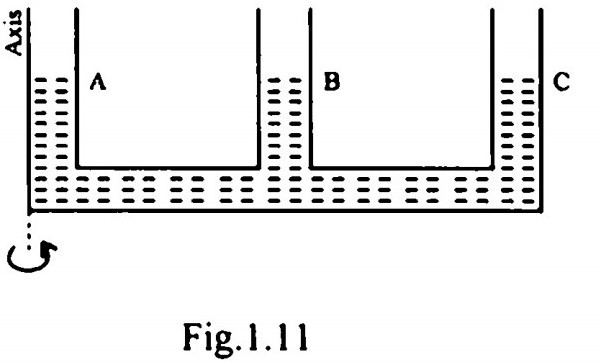Search

# Fluid Mechanics (401-420)

Updated: Apr 23, 2020

401.402.

A. Compressibility- Flight of supersonic aircraft

B. Gravity-Hydraulic jump

C. Viscosity-Flow of real fluid past a tiny sphere

D. Vapour pressure-Cavitation

403. A U-tube manometer is used to measure the pressure in an oil pipe A as shown in Fig. 1.10. The specific gravity of oil is 0.8 and that of mercury is 13.611.76 kN/m²

404. A racing car with a partially filled fuel tank moves in the horizontal direction at a uniform acceleration equal to g. The free surface of the liquid fuel in the tank will assume a slope of

45°

405. A multi-tube manometer filled with water up to level A, B and C as shown in Fig.1.11 is rotated about the vertical axis at AThe water levels at A, B and C will all lie on

a parabola

406. A circular plate of diameter d is submerged in water vertically, so that the

top most point is just at the water surface. The centre of pressure on the plate will be below the water surface at a depth of 5d/8

407. When a ship moving on seawater enters a river and moves inland, it is expected to-sink a little

408.

Submerged body-Force on a curved surface Floating body-Moment of intertia Metacentric height-Metacentre Buoyancy-Force acting vertically up

409. The velocity components in a 2-D flow for an incompressible fluid are given by the equations u = 2xy; v= a² + x² + y² The flow

satisfies irrotationality condition but not the continuity equation

410411 For dynamic similarity between the model and the prototype, the ratio of forces must be the same

412. The limit of the values of the coefficient of discharge of venturi meter is between 0.95 to 0.99

413.Moment of momentum equation-Equation based on conservation of momentum principle applicable to circulatory flows Bernoulli's equation-Equation of motion for onedimensional steady flow of ideal and incompressible fluid Euler's equation-Three dimensional equation of motion based on principle of conservation of momentum for ideal and incompressible fluid flow Hagen Poiseuille equation-Equation to find energy loss in a pipe line having laminar flow

414.Karman vortex street: Flow past a cylinder

415. A pitot tube (coefficient = 1.0) is used to measure the velocity of air of mass density. 1.2 kg/m³. If the head difference in a 14

416417.relation to dimensionless numbers Inertia force is always involved in the

expression of any dimensionless number

Mach number is significant in a flow system where the flow is of

compressible fluid

418. Separation. of boundary layer takes place under

positive pressure gradient in the direction of flow

419. Assertion A : Flow in the boundary layer is always laminar.Reason R : In turbulent flow on a smooth boundary, a laminar sub-layer still exists within the boundary layer. d

420. Assertion A : Gravity influences the pipe flow.

Reason R : Gravity term (g) appears explicitly in Darcy-Weisbach formula for

pipe flow resistance. d